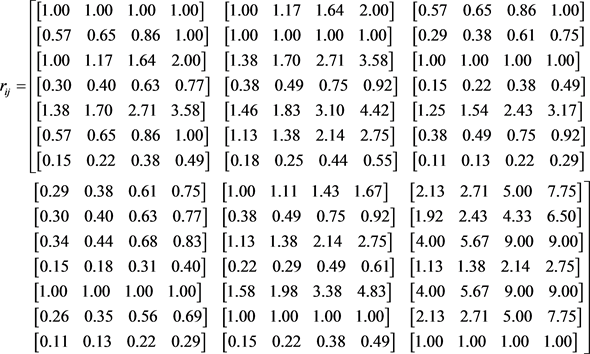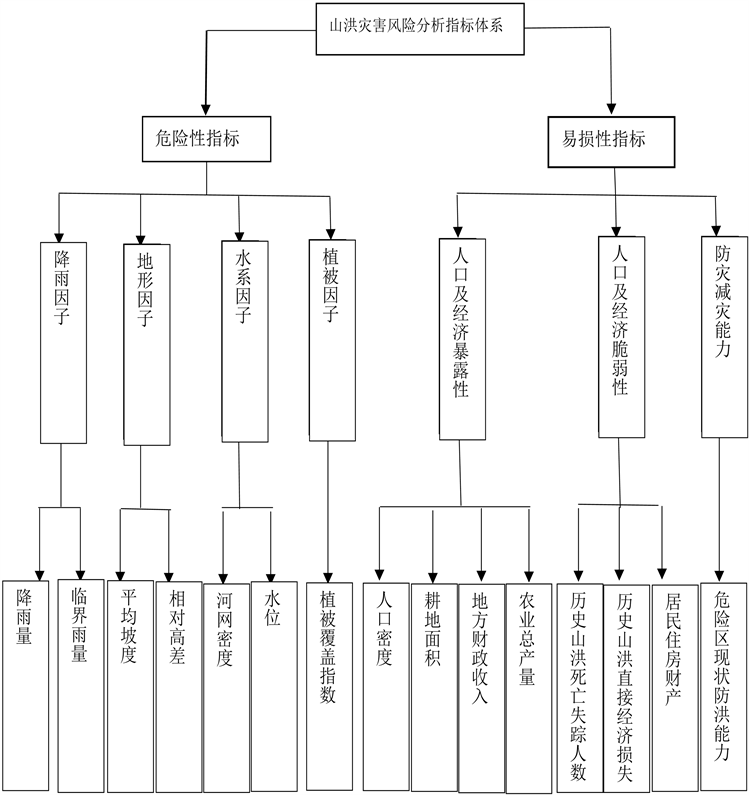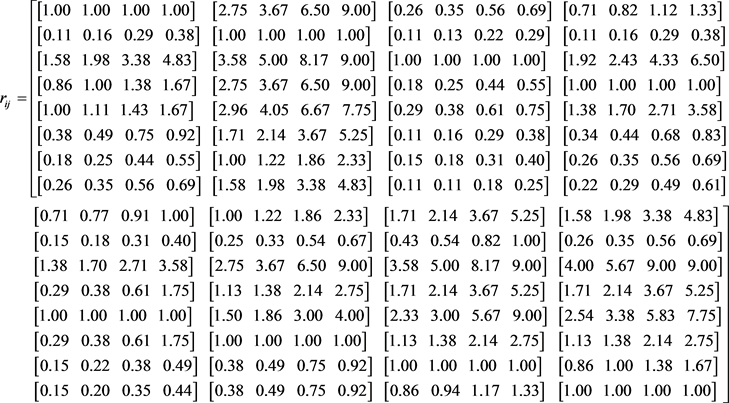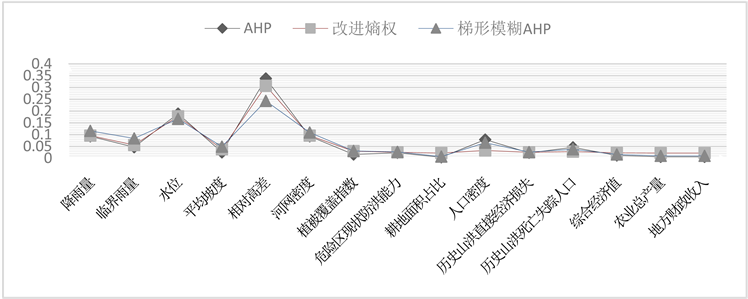﻿ 基于梯形模糊数–灰色聚类的山洪灾害风险区划研究

# 基于梯形模糊数–灰色聚类的山洪灾害风险区划研究Study on Risk Zoning of Mountain Flood Disaster Based on Trapezoidal Fuzzy Number and Grey Clustering

Abstract: In view of the existing risk assessment system of flood disasters in multiplicity, complexity, nonli-nearity and uncertainty, this paper puts forward a comprehensive evaluation model based on trapezoidal fuzzy number and analytic hierarchy process (TFN-AHP), and combined with the grey clustering method, the comprehensive evaluation of the mountain flood disaster area is carried out. In this method, the traditional analytic hierarchy process (AHP) is improved, and the reliability of the evaluation results is improved by accurately quantifying the scale of traditional analytic hierarchy process (AHP). Then, TFN-AHP is applied to the regional risk analysis of mountain tor-rents disaster. The results show that the method is more objective and more practical than the single analytic hierarchy process. Thus, the results of mountain flood risk zoning obtained by this method have high application value in Hubei province.

1. 引言

2. 梯形模糊层次分析法(TFN-AHP)Table 1. Trapezoid fuzzy numbers and corresponding membership functionsTable 2. Importance rank and assignment of elements in comparison with each other

① 综合各专家的偏好信息，其计算公式为：

${r}_{ij}=\left({a}_{ij},{b}_{ij},{c}_{ij},{d}_{ij}\right)=\frac{1}{L}\otimes \left({r}_{ij}^{1}\oplus {r}_{ij}^{1}\oplus \cdots {r}_{ij}^{L}\right)=\left(\frac{1}{L}\underset{k=1}{\overset{L}{\sum }}{a}_{ij}^{\left(k\right)},\frac{1}{L}\underset{k=1}{\overset{L}{\sum }}{b}_{ij}^{\left(k\right)},\frac{1}{L}\underset{k=1}{\overset{L}{\sum }}{c}_{ij}^{\left(k\right)},\frac{1}{L}\underset{k=1}{\overset{L}{\sum }}{d}_{ij}^{\left(k\right)}\right)\text{}\left(i,j\in N\right)$ (1)

${\stackrel{˜}{v}}_{i}=\left({a}_{i}{d}^{-1},{b}_{i}{c}^{-1},{c}_{i}{b}^{-1},{d}_{i}{a}^{-1}\right)$ (2)

② 计算指标Xi的模糊评价值的期望。由于 ${\stackrel{˜}{v}}_{i}$ ( $i\in N$ )为梯形模糊数，先计算出 ${v}_{i}$ 的期望值，即可确定各指标的权重：

$I\left({\stackrel{˜}{v}}_{i}\right)=\alpha {I}^{L}\left({\stackrel{˜}{v}}_{i}\right)+\left(1-\alpha \right){I}^{R}\left({\stackrel{˜}{v}}_{i}\right)$ (3)

$I\left({\stackrel{˜}{v}}_{i}\right)=\frac{{a}_{i}+{b}_{i}+{c}_{i}+{d}_{i}}{4}\text{\hspace{0.17em}}\text{\hspace{0.17em}}\left(i\in N\right)$ (4)

$I\left({\stackrel{˜}{v}}_{i}\right)=\frac{{a}_{i}+2{b}_{i}+{d}_{i}}{4}\text{\hspace{0.17em}}\text{\hspace{0.17em}}\left(i\in N\right)$ (5)

③ 计算指标值的权重。对 $I\left({\stackrel{˜}{v}}_{i}\right)$ 进行归一化即得各指标相对上一层权重为：

${\omega }_{i}=\frac{I\left({\stackrel{˜}{v}}_{i}\right)}{\underset{i=1}{\overset{n}{\sum }}I\left({\stackrel{˜}{v}}_{i}\right)},\text{\hspace{0.17em}}i\in N$ (6)

3. 山洪灾害风险评价方法

3.1. 山洪灾害风险区划评价指标体系

3.2. 计算综合权重Figure 1. Risk assessment index system for mountain torrents3.3. 基于灰色聚类的风险评价

① 计算灰色评价权矩阵。对评价指标 ${x}_{i}\left(i=1,2,3,\cdots ,15\right)$ ，第s个数据属于第e个评价灰类的灰色评价系数记为： ${x}_{ie}^{\left(s\right)}\left(e=1,2,3,4,5\right)$ ，则 ${x}_{ie}^{\left(s\right)}={f}_{ie}\left({x}_{i}\right)$${x}_{i}$ 表示第i个指标值。对评价指标 ${x}_{i}\left(i=1,2,3,\cdots ,15\right)$ ，第s个数据属于各个评价灰类的总灰色评价系数记为 ${x}_{i}^{\left(s\right)}$ ，则有：

${x}_{i}^{\left(s\right)}=\underset{e=1}{\overset{15}{\sum }}{x}_{ie}^{\left(s\right)}$ (7)

${r}_{ie}^{\left(s\right)}=\frac{{x}_{ie}^{\left(s\right)}}{{x}_{i}^{\left(s\right)}}$ (8)

${R}^{\left(s\right)}=\left(\begin{array}{cccc}{r}_{1,1}^{\left(s\right)}& {r}_{1,2}^{\left(s\right)}& \cdots & {r}_{1,5}^{\left(s\right)}\\ {r}_{2,1}^{\left(s\right)}& {r}_{2,2}^{\left(s\right)}& \cdots & {r}_{2,5}^{\left(s\right)}\\ ⋮& ⋮& \ddots & ⋮\\ {r}_{15,1}^{\left(s\right)}& {r}_{15,2}^{\left(s\right)}& \cdots & {r}_{15,5}^{\left(s\right)}\end{array}\right)$ (9)

② 灰色聚类综合评价。对第s个数据作综合评价模型为： ${B}^{\left(s\right)}={U}_{R}\cdot {R}^{\left(s\right)}=\left({b}_{1}^{\left(s\right)},{b}_{2}^{\left(s\right)},\cdots ,{b}_{5}^{\left(s\right)}\right)$ 。若 ${b}_{k}=\mathrm{max}\left\{{b}_{1}^{\left(s\right)},{b}_{2}^{\left(s\right)},\cdots ,{b}_{5}^{\left(s\right)}\right\}$ ，则确定第s个数据属于第k类，进而得到所有数据分类的结果。

4. 实例分析Table 3. Hazard assessment data for mountain torrentsTable 4. Vulnerability assessment data for mountain torrentsTable 5. Risk assessment grading standard

${f}_{1}=\left\{\begin{array}{ll}1\hfill & x\in \left[0,10\right]\hfill \\ \frac{x-40}{-30}\hfill & x\in \left[10,40\right]\hfill \end{array}$ , ${f}_{2}=\left\{\begin{array}{ll}\frac{x-6}{4}\hfill & x\in \left[6,10\right]\hfill \\ 1\hfill & x\in \left[10,70\right]\hfill \\ \frac{x-85}{-15}\hfill & x\in \left[70,85\right]\hfill \end{array}$ , ${f}_{3}=\left\{\begin{array}{ll}\frac{x-40}{30}\hfill & x\in \left[40,70\right]\hfill \\ 1\hfill & x\in \left[70,100\right]\hfill \\ \frac{x-125}{-25}\hfill & x\in \left[100,125\right]\hfill \end{array}$ ,

${f}_{4}=\left\{\begin{array}{ll}\frac{x-85}{15}\hfill & x\in \left[85,100\right]\hfill \\ 1\hfill & x\in \left[100,150\right]\hfill \\ \frac{x-175}{-25}\hfill & x\in \left[150,175\right]\hfill \end{array}$ , ${f}_{5}=\left\{\begin{array}{ll}1\hfill & x\in \left[150,\infty \right]\hfill \\ \frac{x-150}{25}\hfill & x\in \left[125,150\right]\hfill \end{array}$Table 6. Weight coefficient of the evaluation indexesFigure 2. Probability distribution of each indexTable 7. Results of risk assessment grade

5. 结语

 仇蕾, 王慧敏, 马树建. 极端洪水灾害损失评估方法及应用[J]. 水科学进展, 2009, 20(6): 869-875.

 魏一鸣, 金菊良, 杨存建, 等. 洪水灾害风险管理理论[M]. 北京: 科学出版社, 2002: 11-18.

 Zou, Q., Zhou, J.Z., Zhou, C., et al. (2013) Compre-hensive Flood Risk Assessment Based on Set Pair Analysis-Variable Fuzzy Sets Model and Fuzzy AHP. Stochastic Environmental Research and Risk Assessment, 27, 525-546.
https://doi.org/10.1007/s00477-012-0598-5

 Li, Q., Zhou, J.Z., Liu, D.H., et al. (2012) Disaster Risk Assessment Based on Va-riable Fuzzy Sets and Improved Information Diffusion Method. Human and Ecological Risk Assessment, 19, 857-872.
https://doi.org/10.1080/10807039.2012.713824

 赵昱, 刘行, 徐佳欣, 等. 基于层次分析法——灰色聚类的PFI模式下公租房建设的风险评价方法[J]. 工程管理学报, 2013, 27(1): 69-72.

 汪志红, 王斌会. 投影寻踪技术在突发事件风险分类评级中的应用——以广东省雷电灾害风险评价为例[J]. 灾害学, 2011, 26(3): 78-82.

 徐建新, 闫旖君. 基于变异系数法的灰色关联决策模型在城市供水方案优选中应用[J]. 水资源与水工程学报, 2007, 18(4): 9-11.

 欧阳森, 石怡理. 改进熵权法及其在电能质量评估中的应用[J]. 电力系统自动化, 2013, 37(21): 156-159.

 李英海, 周建中. 基于改进熵权和Vague集的多目标防洪调度决策方法[J]. 水电能源学, 2010, 28(6): 32-35.

 罗志猛, 周建中, 杨俊杰, 等. 基于模糊AHP的虚拟研究中心合作伙伴选择[J]. 华中科技大学学报: 自然科学版, 2008, 36(12): 100-103.

 刘雨华. 江苏省沿江开发综合评价研究[D]: [硕士学位论文]. 南京: 南京信息工程大学, 2006: 21-25.

 刘雨华. 基于梯形模糊数的指标权重确定方法的应用研究[J]. 南京信息工程大学学报: 自然科学版, 2009, 1(4): 369-372.

 牟琼, 杨春德. 一种基于梯形模糊数互补判断矩阵确定权重的方法[J]. 重庆邮电学院学报: 自然科学版, 2006, 18(6): 809-812.

 王正新, 党耀国, 裴玲玲. 基于相对隶属度的灰色聚类评估方法[J]. 统计与决策, 2012(3): 100-102.

 杨秀文, 付诗禄, 顾又川, 等. 两类白化权函数的比较[J]. 后勤工程学院学报, 2010, 26(1): 88-91.

 丁文峰, 杜俊, 陈小平, 等. 四川省山洪灾害风险评估与区划[J]. 长江科学院院报, 2015, 32(12): 41-45.

 邹强, 周建中, 杨小玲, 等. 属性区间识别模型在溃坝后果综合评价中的应用[J]. 四川大学学报: 工程科学版, 2011, 43(2): 45-50.

Top